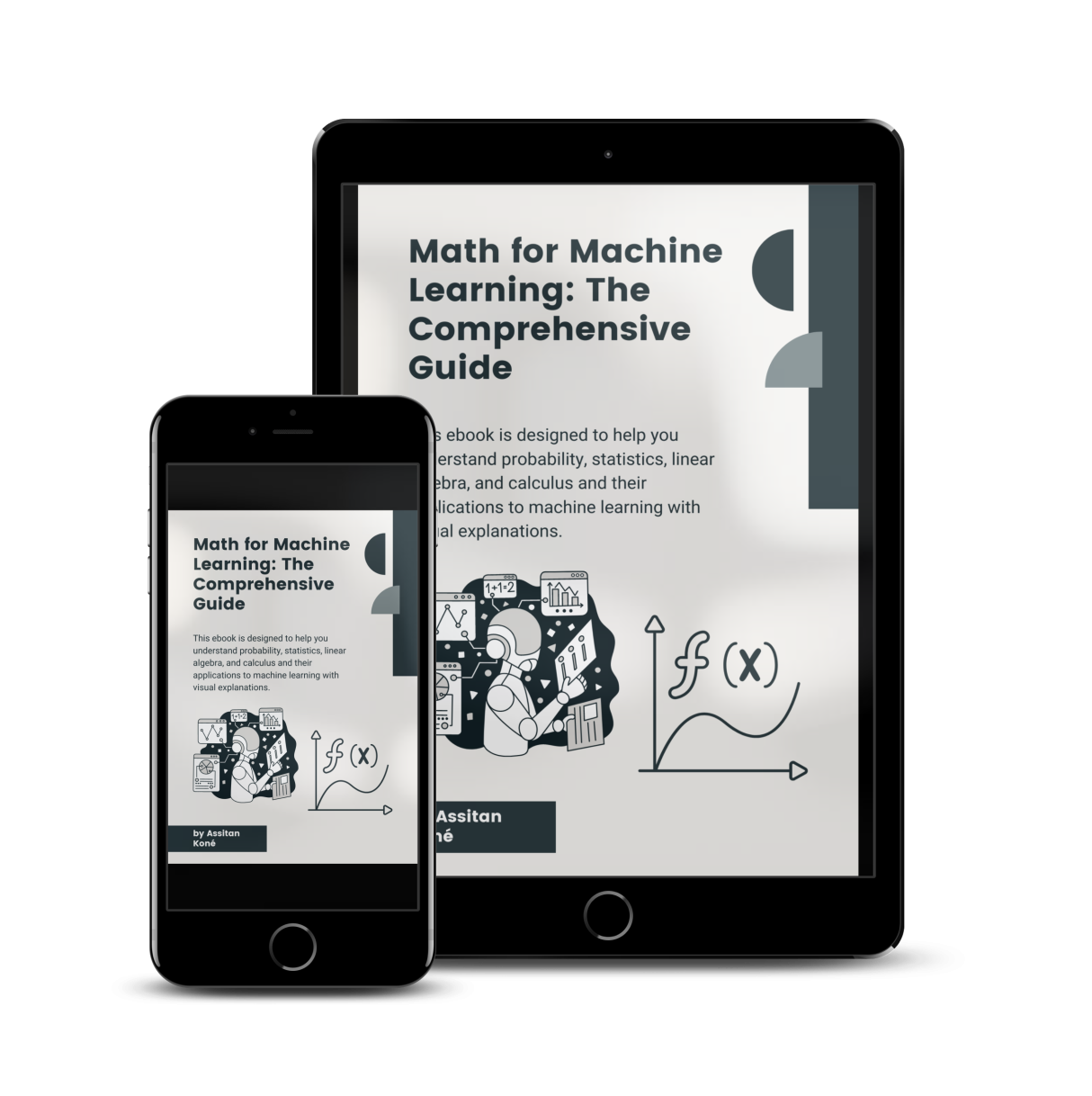# The Ultimate guide to understanding Math for Machine Learning

Grasp fundamentals with engaging illustrations so you can understand quickly the concepts.

## Understand why Math is important to do machine learning.

• Learn with simple illustrations what are probabilities, statistics, linear algebra and calculus
• Discover the applications of these fields in machine learning

# 01

### Foundations of mathematics

Presentation of some symbols used in machine learning.

# 02

### Probabilities

Vectors and matrices explained visually.

# 03

### Statistics

Why derivatives are important in machine learning and what is the application with gradient descent.

# 04

### Linear algebra

Vectors and matrices explained visually.

# 05

### Calculus

Why derivatives are important in machine learning and what is the application with gradient descent.## Download our free Math for Data Science guide now and start learning!

Don't miss out on the opportunity to learn the fundamentals of Math for Data Science with this small guide.